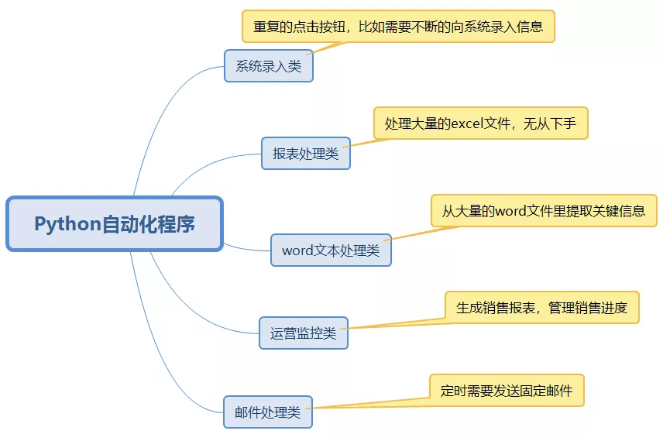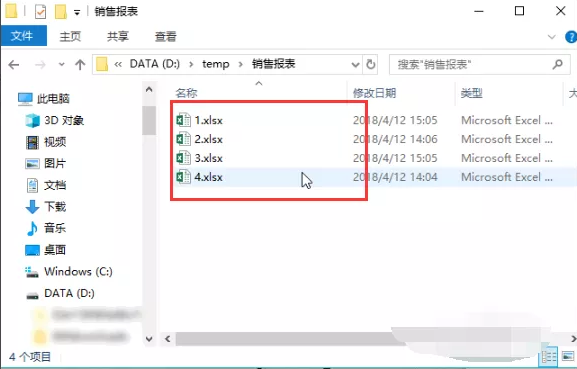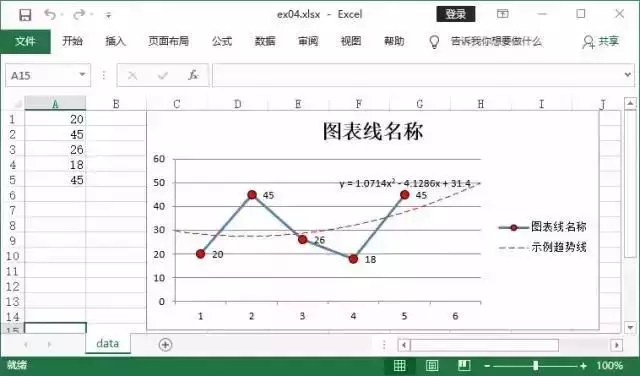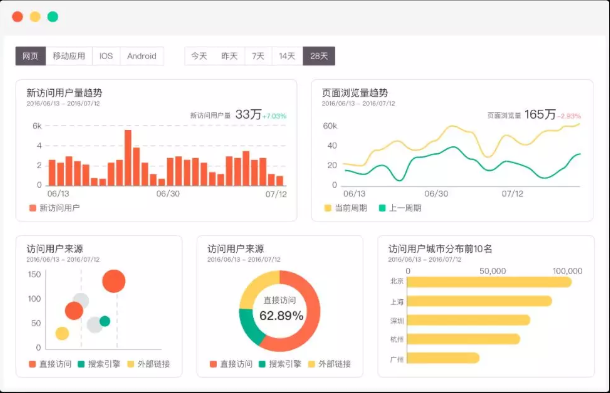# 学了这些Python“偷懒”技巧，工作再也不累了• 爱喝马黛茶的安东尼   2019-12-10 15:03:101092`pip install splinter`

```#coding=utf-8
import time
from splinter import Browser
def splinter(url):
browser = Browser()
browser.visit(url)
time.sleep(5)
browser.find_by_id('idInput').fill('xxxxxx')
browser.find_by_id('pwdInput').fill('xxxxx')
time.sleep(8)
#close the window of brower
browser.quit()
if __name__ == '__main__':
websize = 'https://mail.163.com/'
splinter(websize)```

```import win32api
import time
def move_click(x, y, t=0):  # 移动鼠标并点击左键
win32api.SetCursorPos((x, y))  # 设置鼠标位置(x, y)
win32api.mouse_event(win32con.MOUSEEVENTF_LEFTDOWN |
win32con.MOUSEEVENTF_LEFTUP, x, y, 0, 0)  # 点击鼠标左键
if t == 0:
time.sleep(random.random()*2+1)  # sleep一下
else:
time.sleep(t)
return 0
# 测试
move_click(30, 30)
def resolution():  # 获取屏幕分辨率
return win32api.GetSystemMetrics(0), win32api.GetSystemMetrics(1)```

https://github.com/JamesRaynor67/jump

Excel自动化处理

Excel合并```# -*- coding: utf-8 -*-
#将多个Excel文件合并成一个
import xlrd
import xlsxwriter
#获取excel中所有的sheet表
def getsheet(fh):
return fh.sheets()
#获取sheet表的行数
def getnrows(fh,sheet):
table=fh.sheets()[sheet]
return table.nrows
#读取文件内容并返回行内容
def getFilect(file,shnum):
fh=open_xls(file)
table=fh.sheets()[shnum]
num=table.nrows
for row in range(num):
rdata=table.row_values(row)
datavalue.append(rdata)
return datavalue```

```for i in var_list:
df_0 = data[['var_1','var_2','var_3','var_4',i]][data[i]=='信息']
df_0['month'] = date_replace(i)
df_0 = df_0[['var_1','var_2','var_3','var_4','var_5']]
li.append(df_0)
writer = pd.ExcelWriter(r'C:\Users\mapping.xlsx')
df = pd.concat(li)
df```

Excel中添加数据图表

```import xlsxwriter
#设置一个例子
data = [20, 45, 26, 18, 45]
#创建表格
workbook = xlsxwriter.Workbook("temp.xlsx")
#添加数据
worksheet.write_column('A1', data)
#创建图表
#图表添加数据
'values': '=data!\$A1:\$A6',
'name': '图表名称',
'marker': {
'type': 'circle',
'size': 8,
'border': {'color': 'black'},
'fill': {'color': 'red'}
} ,
'data_labels': {'values': True},
'trendline': {
'type': 'polynomial',
'order': 2,
'name': '趋势线',
'forward': 0.5,
'backward': 0.5,
'display_equation':True,
'line': {'color': 'red', 'width':1, 'dash_type': 'long_dash'}
}
})
worksheet.insert_chart('c1', chart)
workbook.close()```word关键信息提取docx文件自己本身是压缩文件，打开压缩包之后竟然发现里面有个专门存储word里面文本的文件。

1. 打开docx的压缩包

2. 获取word里面的正文信息

3. 利用正则表达式匹配出我们想要的信息

4. 将信息存储到txt中（txt可以用excel打开）

5. 批量调用上述过程，完成一万份简历的提取工作

```import re
def get_field_value(text):
value_list = []
m = re.findall(r"姓名(.*?)性别", table)
value_list.append(m)
m = re.findall(r"性别(.*?)学历", table)
value_list.append(m)
m = re.findall(r"民族(.*?)健康状况", table)
value_list.append(m)
'''
此处省略其他字段匹配
'''
return value_list```

https://blog.csdn.net/geoker/article/details/801494631.利用python操作线下文件将其载入数据库

2.通过数据库对数据进行处理

3.利用python输出结果

```from impala.dbapi import connect
from impala.util import as_pandas
import datetime
#host：数据库域名
#user：数据库用户名
rows =[]
for index, row in df_data.iterrows():
rows.append('('+'"'+str(row['case_id']).replace('nan','null')+'"'+','+'"'+str(row['birth_date'])+'"'+')'+',')
a= '''
INSERT into table
(case_id, birth_date)
values '''
for i in rows:
a += i
a = a[:-1]
cursor1 = conn.cursor()
cursor1.execute(a)
cursor1.close()
conn.close()
print('成功导入数据至数据库...')
del a
del rows```

1、直接利用python链接数据库进行一些列的操作

2、导出你所需要的结果

```import sql   #sql是封装的sql文件
sql_end = sql.sql_end
cursor1 = conn.cursor()
for i in sql_end.split(';'):
print(i)
cursor1.execute(i)
cursor1.close()
conn.close()
print('程序运行结束，请执行下一步。')```

python连接数据库：

https://blog.csdn.net/weixin_42213622/article/details/86523400

"Talk is cheap, show you the code"

1、正文

2、图片

3、附件

OK

```from email.mime.text import MIMEText
from email.mime.multipart import MIMEMultipart
from email.mime.image import MIMEImage
import smtplib
msg = MIMEMultipart()```

```##在邮件中插入文本信息
df_text='''<html>
<body>
<p>   Hi all ，</p>
<p>   这是一个测试邮件，详情请参考附件 </p>
<p>   情况如下图： </p>
</body></html>'''
msgtext = MIMEText(df_text, 'html', 'utf-8')
msg.attach(msgtext)```

```##在邮件中插入图片信息
image = open('temp.jpg','rb')
msg.attach(msgimage)```

```##在邮件添加附件
msgfile = MIMEText(open('temp.xlsx', 'rb').read(), 'base64', 'utf-8')
msgfile["Content-Disposition"] = 'attachment; filename="temp.xlsx"'
msg.attach(msgfile)```

```#设置邮件信息常量
email_host= ''  # 服务器地址
sender = '' # 发件人

```try:
smtp = smtplib.SMTP(host=email_host)
smtp.connect(email_host)
smtp.starttls()
smtp.quit()
print('发送成功')
except Exception:
print('发送失败')```python学习网，免费的在线学习python平台，欢迎关注！